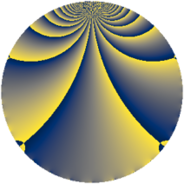# Properties

 Label 2760.2.tLevel $2760$ Weight $2$ Character orbit 2760.t Rep. character $\chi_{2760}(1381,\cdot)$ Character field $\Q$ Dimension $176$ Sturm bound $1152$

# Related objects

## Defining parameters

 Level: $$N$$ $$=$$ $$2760 = 2^{3} \cdot 3 \cdot 5 \cdot 23$$ Weight: $$k$$ $$=$$ $$2$$ Character orbit: $$[\chi]$$ $$=$$ 2760.t (of order $$2$$ and degree $$1$$) Character conductor: $$\operatorname{cond}(\chi)$$ $$=$$ $$8$$ Character field: $$\Q$$ Sturm bound: $$1152$$

## Dimensions

The following table gives the dimensions of various subspaces of $$M_{2}(2760, [\chi])$$.

Total New Old
Modular forms 584 176 408
Cusp forms 568 176 392
Eisenstein series 16 0 16

## Trace form

 $$176q - 176q^{9} + O(q^{10})$$ $$176q - 176q^{9} + 16q^{14} - 16q^{16} + 16q^{20} + 24q^{22} - 24q^{24} - 176q^{25} - 16q^{26} + 24q^{28} + 40q^{32} - 40q^{38} - 24q^{42} - 24q^{44} + 144q^{49} - 16q^{52} - 32q^{55} + 40q^{56} + 32q^{57} + 56q^{58} - 96q^{62} + 24q^{66} - 56q^{68} - 8q^{70} + 64q^{71} + 32q^{73} + 40q^{74} - 120q^{76} - 16q^{78} + 176q^{81} + 40q^{82} - 24q^{84} - 40q^{86} - 48q^{87} + 80q^{88} + 40q^{96} - 32q^{97} + 56q^{98} + O(q^{100})$$

## Decomposition of $$S_{2}^{\mathrm{new}}(2760, [\chi])$$ into newform subspaces

The newforms in this space have not yet been added to the LMFDB.

## Decomposition of $$S_{2}^{\mathrm{old}}(2760, [\chi])$$ into lower level spaces

$$S_{2}^{\mathrm{old}}(2760, [\chi]) \cong$$ $$S_{2}^{\mathrm{new}}(24, [\chi])$$$$^{\oplus 4}$$$$\oplus$$$$S_{2}^{\mathrm{new}}(40, [\chi])$$$$^{\oplus 4}$$$$\oplus$$$$S_{2}^{\mathrm{new}}(120, [\chi])$$$$^{\oplus 2}$$$$\oplus$$$$S_{2}^{\mathrm{new}}(184, [\chi])$$$$^{\oplus 4}$$$$\oplus$$$$S_{2}^{\mathrm{new}}(552, [\chi])$$$$^{\oplus 2}$$$$\oplus$$$$S_{2}^{\mathrm{new}}(920, [\chi])$$$$^{\oplus 2}$$